# Imbedding of rings

(diff) ← Older revision | Latest revision (diff) | Newer revision → (diff)

A monomorphism of a ring into another ring; a ringis imbeddable in a ringifis isomorphic to a subring of. The conditions for imbedding of an associative ring in an (associative) skew-field and of an arbitrary ring into a division ring have been studied in great detail. These studies were initiated by A.I. Mal'tsev , who constructed an example of an associative ring without zero divisors and not imbeddable in a skew-field. The following Mal'tsev problem remained an open question for a long time: Is any associative ring without zero divisors and for which the semi-group of non-zero elements is imbeddable in a group, imbeddable in a skew-field? This problem was negatively answered in 1966 (cf. ). A square matrixof order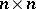over an associative ringis said to be non-full if it can be represented in the form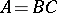where,are matrices of ordersand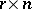, respectively, and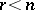. Letbe square matrices of orderoverin which all columns (except, possibly, the first column) are identical. Then the matrix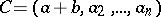is said to be the determinant sum ofandwith respect to the first column. The determinant sum of square matrices of the same order with respect to an arbitrary column (row) is defined in a similar manner. An associative ringwith a unit element is imbeddable in a skew-field if and only if it has no zero divisors and if no scalar matrix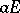with a non-zero elementalong the diagonal can be represented as the determinant sum of a finite number of non-full matrices . The class of associative rings imbeddable in skew-fields is not finitely axiomatized (i.e. cannot be defined by a finite number of axioms) . A number of sufficient conditions for imbedding of an associative ring in a skew-field are known; the following are the most important. Letbe an associative ring without zero divisors and for which the semi-group of non-zero elements satisfies Ore's condition (cf. Imbedding of semi-groups). Thenis imbeddable in a skew-field . The group algebra of an ordered group is imbeddable in a skew-field (the Mal'tsev–Neumann theorem, cf. ). An arbitrary domain of free right (left) ideals (cf. Associative rings and algebras) is imbeddable in a skew-field .

A ringis imbeddable in a division ring if and only if it has no zero divisors. Let,be rings, letbe a symbol,. A mapping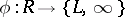is said to be a-homomorphism if: 1) the set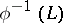is a ring and the mappingon this set is a ring homomorphism; 2) it follows from,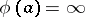that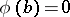; and 3) it follows from,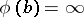that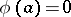. A-homomorphism of a field is nothing but a specialization (of a point) of the field (cf. Specialization of a point). A division ringis a free-extension of a ringifincludesand is generated (as a division ring) by the ring, while any-homomorphism of the ringinto some division ringmay be extended to a-homomorphism ofinto. Every ring without zero divisors has a free-extension .

How to Cite This Entry:
Imbedding of rings. Encyclopedia of Mathematics. URL: http://encyclopediaofmath.org/index.php?title=Imbedding_of_rings&oldid=19119
This article was adapted from an original article by L.A. Bokut' (originator), which appeared in Encyclopedia of Mathematics - ISBN 1402006098. See original article